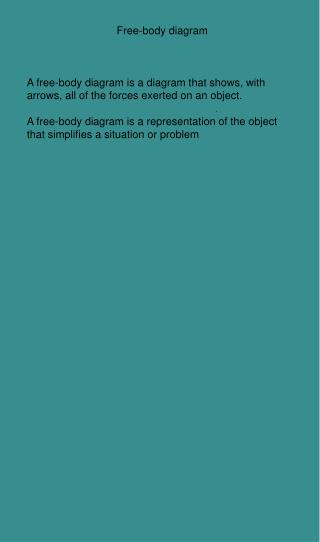Download PresentationFree-body diagram

# Free-body diagram

Télécharger la présentation## Free-body diagram

- - - - - - - - - - - - - - - - - - - - - - - - - - - E N D - - - - - - - - - - - - - - - - - - - - - - - - - - -
##### Presentation Transcript

1. Free-body diagram A free-body diagram is a diagram that shows, with arrows, all of the forces exerted on an object. A free-body diagram is a representation of the object that simplifies a situation or problem

2. Free-body diagram Method: 1. Determine the body whose situation is to be analyzed 2. Represent the body with a point or square that corresponds to the object's centre 3. Show, with arrows, all of the forces acting on the body, making the origin of each arrow coincide with the point (square) drawn in the previous step; the length of the arrows must be proportional to the magnitude of the forces illustrated.(draw them to scale)

3. Free-body diagram A box has a mass of 12kg Fg =9.8m/s2 x 12kg = 117.6N directed down The table it sits on exerts a normal force up, equal to the force of gravity A student pushes with a force of 40N to the right The force of friction is 50N to the left

4. Free body diagram 1) Represent the body/object with a point that corresponds to the object's center 2) Show with arrows, all of the forces acting on the body. The tail of the vector should start at the point the length of the vector is proportional to the magnitude of the vector (use a ruler) 3) Set the origin of the Cartesian plane at the point, the y axis coincides with the normal(when applicable)

5. Page 289 Draw free body diagrams

6. Resultant Force Remember that forces of vectors If the vectors are at an angle we must decompose them (like we did with initial velocity) CAREFUL: Is the angle from the horizontal (x axis) or the vertical (y axis). ? Adjust accordingly. This vector is vertical No need to decompose This vector is at an angle X component = y component = FN = 30 N FT = 20N 500 Fg = 30N This vector is vertical No need to decompose

7. Once all the forces are decomposed Add up all the x components Add up all the y components Determine the resultant vector Magnitude: Pythagoras Angle: Trig Example B page 291 Find the magnitude of FR

8. Once all the forces are decomposed Add up all the x components Add up all the y components Determine the resultant vector Magnitude: Pythagorus Angle: Trig Example B page 291 Find the magnitude of FR

9. Equilibrium. Determine the Resultant force acting on a body. (Add up all the vector components) To put the body into equilibrium: add a new force equal in magnitude but opposite in direction (180o in a new direction) F = 120N [E49oN] To put the system in equlibrium Add a Force that is 120N [W49oS]

10. Example C If given a cartesian plane, use the graph paper to calculate the x and y coponents (easy) FR= θ

11. Example C If given a cartesian plane, use the graph paper to calculate the x and y coponents (easy) 64N FR= 64 141 +50 θ=tan-1 (40/50) = 39o θ -40 The angle in trig angle method is 141o So to put the system into equilibrium, I will add a vector of 64N at an angle of 141o + 180o = 321o

12. Section 13.2/ p. 292

13. Equilibrium When FR = 0

14. When we add up all the x components they = 0 When we add up all the y components they = 0 then we can say the body is in a state of equilibrium

15. Static Equilibrium: the object is stationary Dynamic Equilibrium: the object is at a constant velocity (and FR = 0)

16. Types of questions: Verify if the body is in equilibrium What force can we add to put the body into equilibrium FR = 0 N Feq = -FR we add up all the Forces (FR), the Force (Feq) required will be the same magnitude as FR but opposite direction